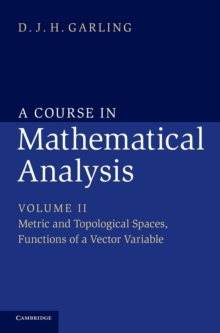Supporting your high street Find out how »
• My AccountBasket# A A Course in Mathematical Analysis 3 Volume Set A Course in Mathematical Analysis : Metric and Topological Spaces, Functions of a Vector Variable Volume 2 Hardback

#### Description

The three volumes of A Course in Mathematical Analysis provide a full and detailed account of all those elements of real and complex analysis that an undergraduate mathematics student can expect to encounter in their first two or three years of study.

Containing hundreds of exercises, examples and applications, these books will become an invaluable resource for both students and teachers.

Volume 1 focuses on the analysis of real-valued functions of a real variable.

This second volume goes on to consider metric and topological spaces.

Topics such as completeness, compactness and connectedness are developed, with emphasis on their applications to analysis.

This leads to the theory of functions of several variables.

Differential manifolds in Euclidean space are introduced in a final chapter, which includes an account of Lagrange multipliers and a detailed proof of the divergence theorem.

Volume 3 covers complex analysis and the theory of measure and integration.

#### Information

• Format: Hardback
• Pages: 336 pages, Worked examples or Exercises; 15 Line drawings, unspecified
• Publisher: Cambridge University Press
• Publication Date:
• Category: Calculus & mathematical analysis
• ISBN: 9781107032033

£82.00

£71.89

on all orders

###### Pick up orders

from local bookshops